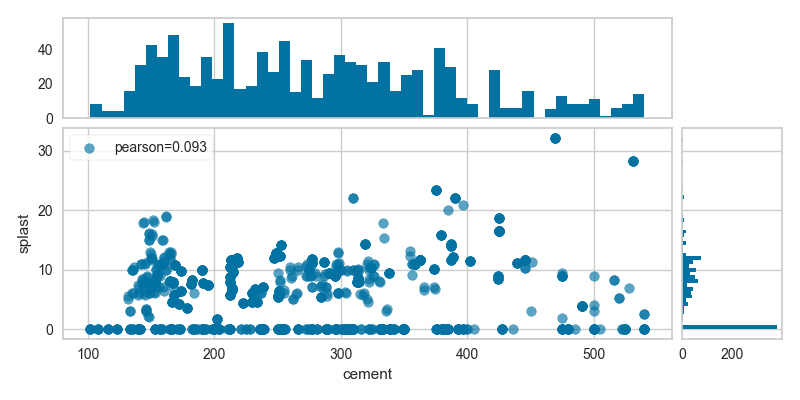# Direct Data Visualization¶

Sometimes for feature analysis you simply need a scatter plot to determine the distribution of data. Machine learning operates on high dimensional data, so the number of dimensions has to be filtered. As a result these visualizations are typically used as the base for larger visualizers; however you can also use them to quickly plot data during ML analysis.

## Joint Plot Visualization¶

A joint plot visualizer plots a feature against the target and shows the distribution of each via a histogram on each axis.

# Load the data
feature = "cement"
target = "strength"

# Get the X and y data from the DataFrame
X = df[feature]
y = df[target]

from yellowbrick.features import JointPlotVisualizer

visualizer = JointPlotVisualizer(feature=feature, target=target)

visualizer.fit(X, y)
visualizer.poof()The joint plot visualizer can also be plotted with hexbins in the case of many, many points.

visualizer = JointPlotVisualizer(
feature=feature, target=target, joint_plot='hex'
)

visualizer.fit(X, y)
visualizer.poof()## API Reference¶

class yellowbrick.features.jointplot.JointPlotVisualizer(ax=None, feature=None, target=None, joint_plot='scatter', joint_args=None, xy_plot='hist', xy_args=None, size=600, ratio=5, space=0.2, **kwargs)[source]

Bases: yellowbrick.features.base.FeatureVisualizer

JointPlotVisualizer allows for a simultaneous visualization of the relationship between two variables and the distrbution of each individual variable. The relationship is plotted along the joint axis and univariate distributions are plotted on top of the x axis and to the right of the y axis.

Parameters:
ax: matplotlib Axes, default: None

This is inherited from FeatureVisualizer but is defined within JointPlotVisualizer since there are three axes objects.

feature: string, default: None

The name of the X variable If a DataFrame is passed to fit and feature is None, feature is selected as the column of the DataFrame. There must be only one column in the DataFrame.

target: string, default: None

The name of the Y variable If target is None and a y value is passed to fit then the target is selected from the target vector.

joint_plot: one of {‘scatter’, ‘hex’}, default: ‘scatter’

The type of plot to render in the joint axis Currently, the choices are scatter and hex. Use scatter for small datasets and hex for large datasets

joint_args: dict, default: None

Keyword arguments used for customizing the joint plot:

 Property Description alpha transparency facecolor background color of the joint axis aspect aspect ratio fit used if scatter is selected for joint_plot to draw a best fit line - values can be True or False. Uses Yellowbrick.bestfit estimator used if scatter is selected for joint_plot to determine the type of best fit line to use. Refer to Yellowbrick.bestfit for types of estimators that can be used. x_bins used if hex is selected to set the number of bins for the x value y_bins used if hex is selected to set the number of bins for the y value cmap string or matplotlib cmap to colorize lines Use either color to colorize the lines on a per class basis or colormap to color them on a continuous scale.
xy_plot: one of {‘hist’}, default: ‘hist’

The type of plot to render along the x and y axes Currently, the choice is hist

xy_args: dict, default: None

Keyword arguments used for customizing the x and y plots:

 Property Description alpha transparency facecolor_x background color of the x axis facecolor_y background color of the y axis bins used to set up the number of bins for the hist plot histcolor_x used to set the color for the histogram on the x axis histcolor_y used to set the color for the histogram on the y axis
size: float, default: 600

Size of each side of the figure in pixels

ratio: float, default: 5

Ratio of joint axis size to the x and y axes height

space: float, default: 0.2

Space between the joint axis and the x and y axes

kwargs : dict

Keyword arguments that are passed to the base class and may influence the visualization as defined in other Visualizers.

Notes

These parameters can be influenced later on in the visualization process, but can and should be set as early as possible.

Examples

>>> visualizer = JointPlotVisualizer()
>>> visualizer.fit(X,y)
>>> visualizer.poof()

draw(X, y, **kwargs)[source]

Sets up the layout for the joint plot draw calls draw_joint and draw_xy to render the visualizations.

draw_joint(X, y, **kwargs)[source]

Draws the visualization for the joint axis.

draw_xy(X, y, **kwargs)[source]

Draws the visualization for the x and y axes

finalize(**kwargs)[source]

Finalize executes any subclass-specific axes finalization steps. The user calls poof and poof calls finalize.

Parameters: kwargs: generic keyword arguments.
fit(X, y, **kwargs)[source]

Sets up the X and y variables for the jointplot and checks to ensure that X and y are of the correct data type

Fit calls draw

Parameters: X : ndarray or DataFrame of shape n x 1 A matrix of n instances with 1 feature y : ndarray or Series of length n An array or series of the target value kwargs: dict keyword arguments passed to Scikit-Learn API.